# Basic barplot with ggplot2

This post explains how to draw barplots with `R` and ggplot2, using the `geom_bar()` function. It starts with the most basic example and describes a few possible customizations. If you’re not familiar with ggplot2 at all, try this course as an introduction.

# Most basic barplot with `geom_bar()`

This is the most basic barplot you can build using the ggplot2 package. It follows those steps:

• always start by calling the `ggplot()` function.
• then specify the `data` object. It has to be a data frame. And it needs one numeric and one categorical variable.
• then come thes aesthetics, set in the `aes()` function: set the categoric variable for the X axis, use the numeric for the Y axis
• finally call `geom_bar()`. You have to specify `stat="identity"` for this kind of dataset.``````# Load ggplot2
library(ggplot2)

# Create data
data <- data.frame(
name=c("A","B","C","D","E") ,
value=c(3,12,5,18,45)
)

# Barplot
ggplot(data, aes(x=name, y=value)) +
geom_bar(stat = "identity")``````

# Control bar color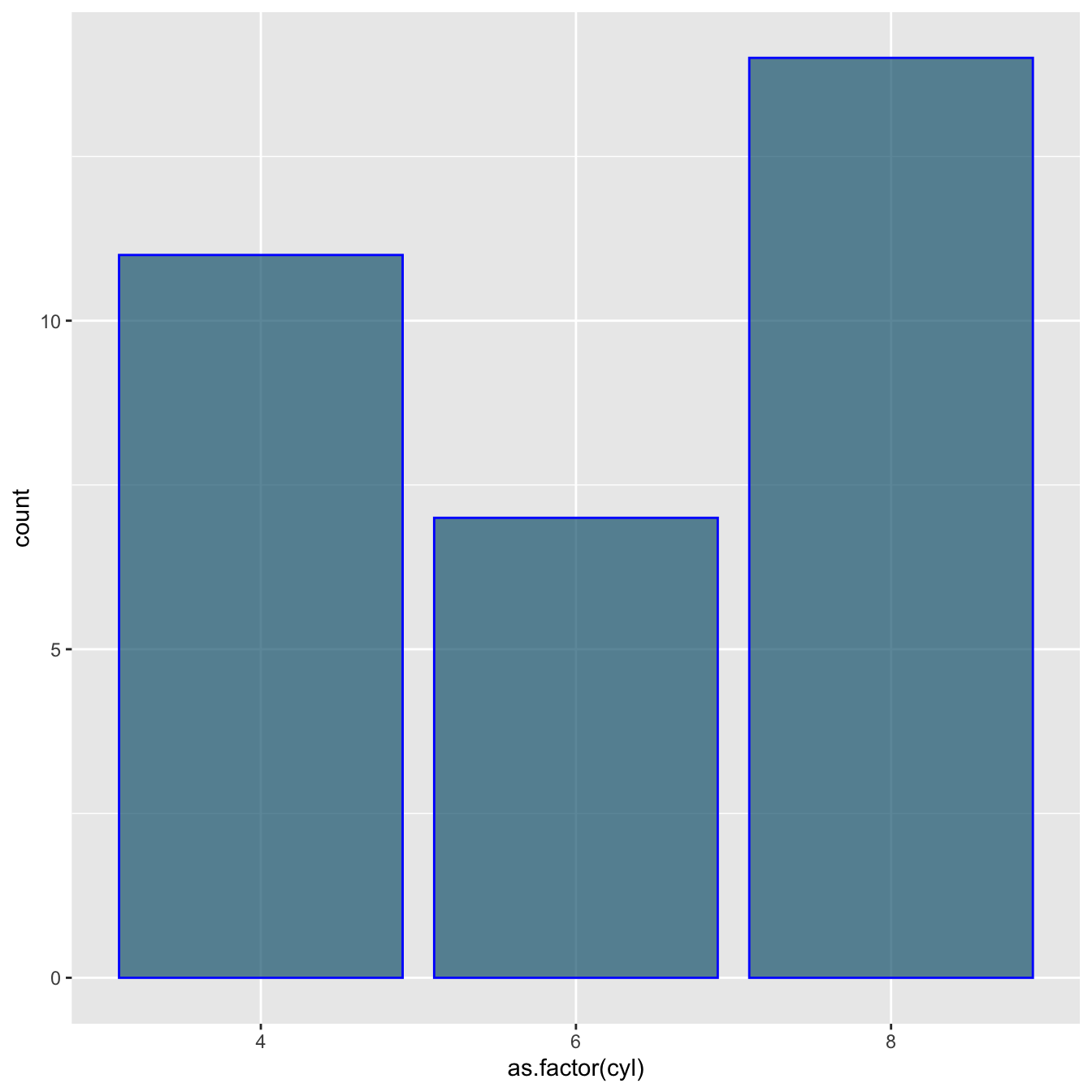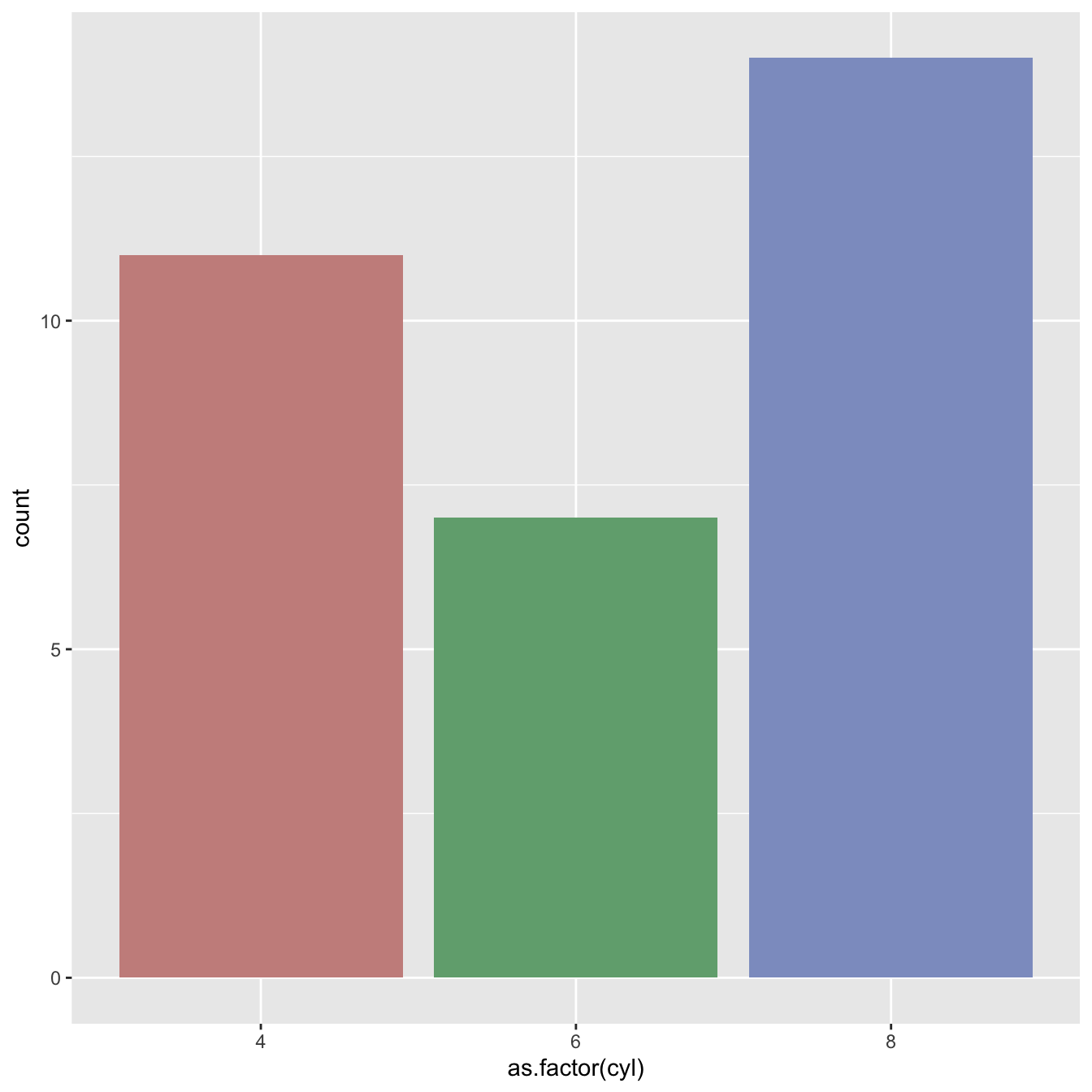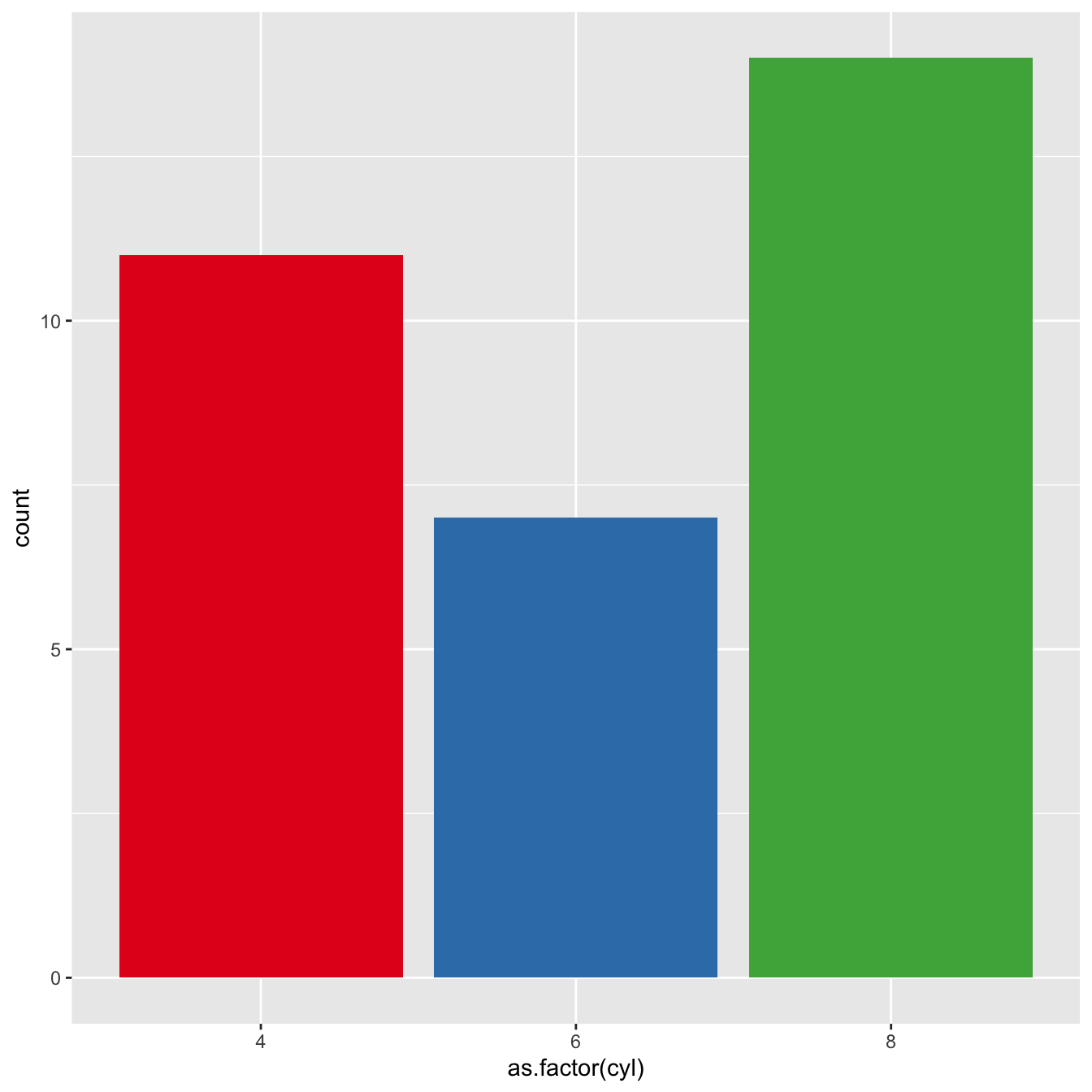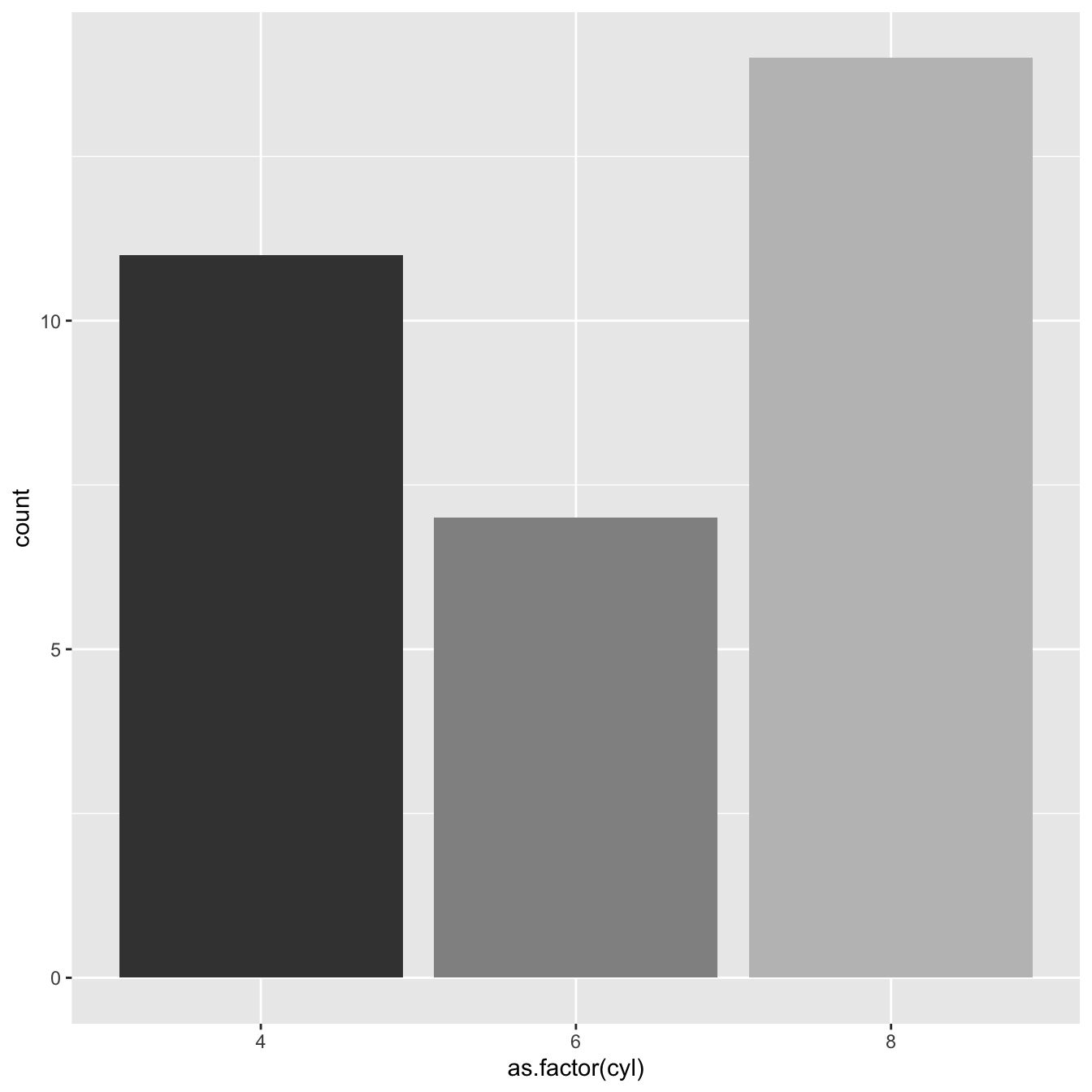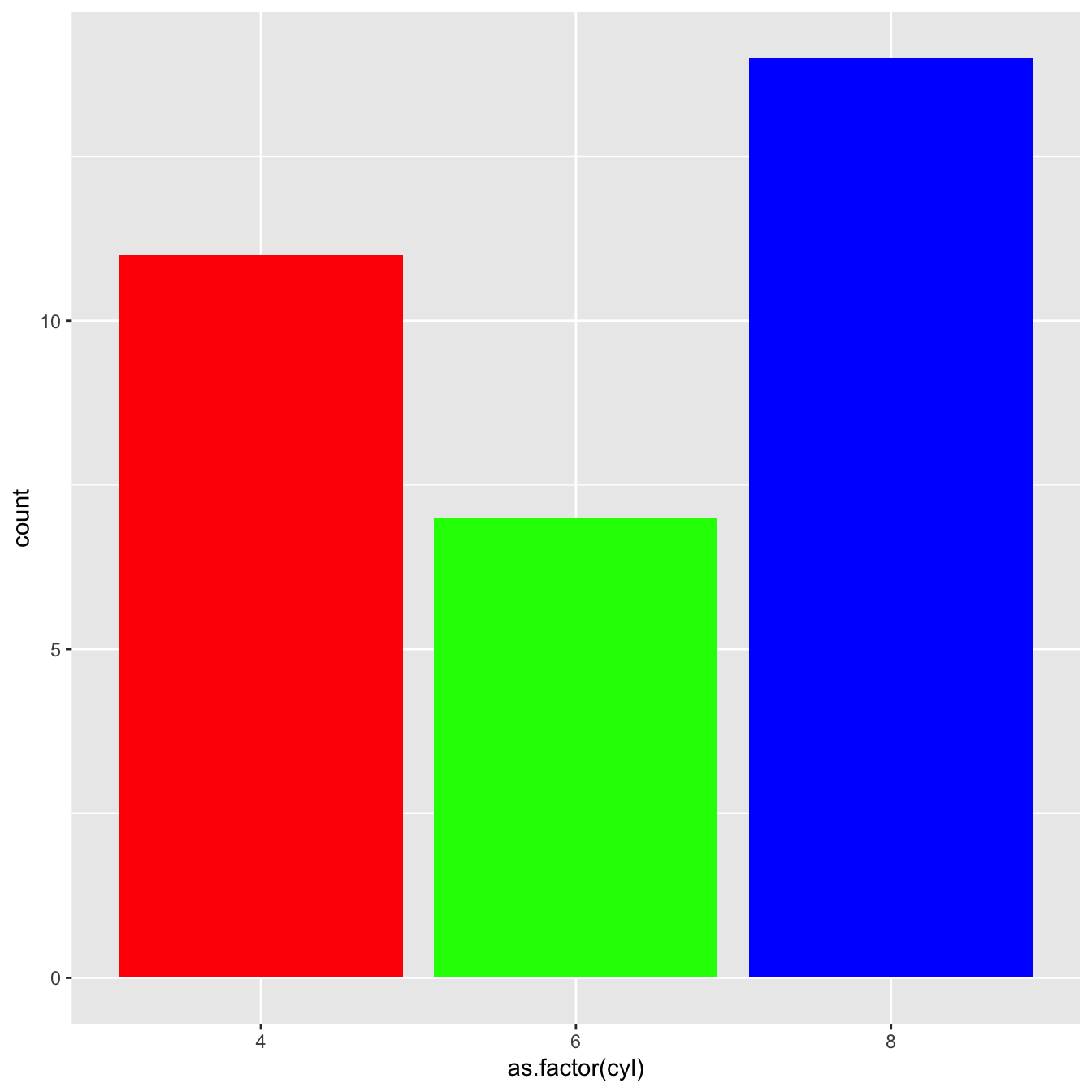Here are a few different methods to control bar colors. Note that using a legend in this case is not necessary since names are already displayed on the X axis. You can remove it with `theme(legend.position="none")`.

``````# Libraries
library(ggplot2)

# 1: uniform color. Color is for the border, fill is for the inside
ggplot(mtcars, aes(x=as.factor(cyl) )) +
geom_bar(color="blue", fill=rgb(0.1,0.4,0.5,0.7) )

# 2: Using Hue
ggplot(mtcars, aes(x=as.factor(cyl), fill=as.factor(cyl) )) +
geom_bar( ) +
scale_fill_hue(c = 40) +
theme(legend.position="none")

# 3: Using RColorBrewer
ggplot(mtcars, aes(x=as.factor(cyl), fill=as.factor(cyl) )) +
geom_bar( ) +
scale_fill_brewer(palette = "Set1") +
theme(legend.position="none")

# 4: Using greyscale:
ggplot(mtcars, aes(x=as.factor(cyl), fill=as.factor(cyl) )) +
geom_bar( ) +
scale_fill_grey(start = 0.25, end = 0.75) +
theme(legend.position="none")

# 5: Set manualy
ggplot(mtcars, aes(x=as.factor(cyl), fill=as.factor(cyl) )) +
geom_bar( ) +
scale_fill_manual(values = c("red", "green", "blue") ) +
theme(legend.position="none")``````

# Horizontal barplot with `coord_flip()`

It often makes sense to turn your barplot horizontal. Indeed, it makes the group labels much easier to read.

Fortunately, the `coord_flip()` function makes it a breeze.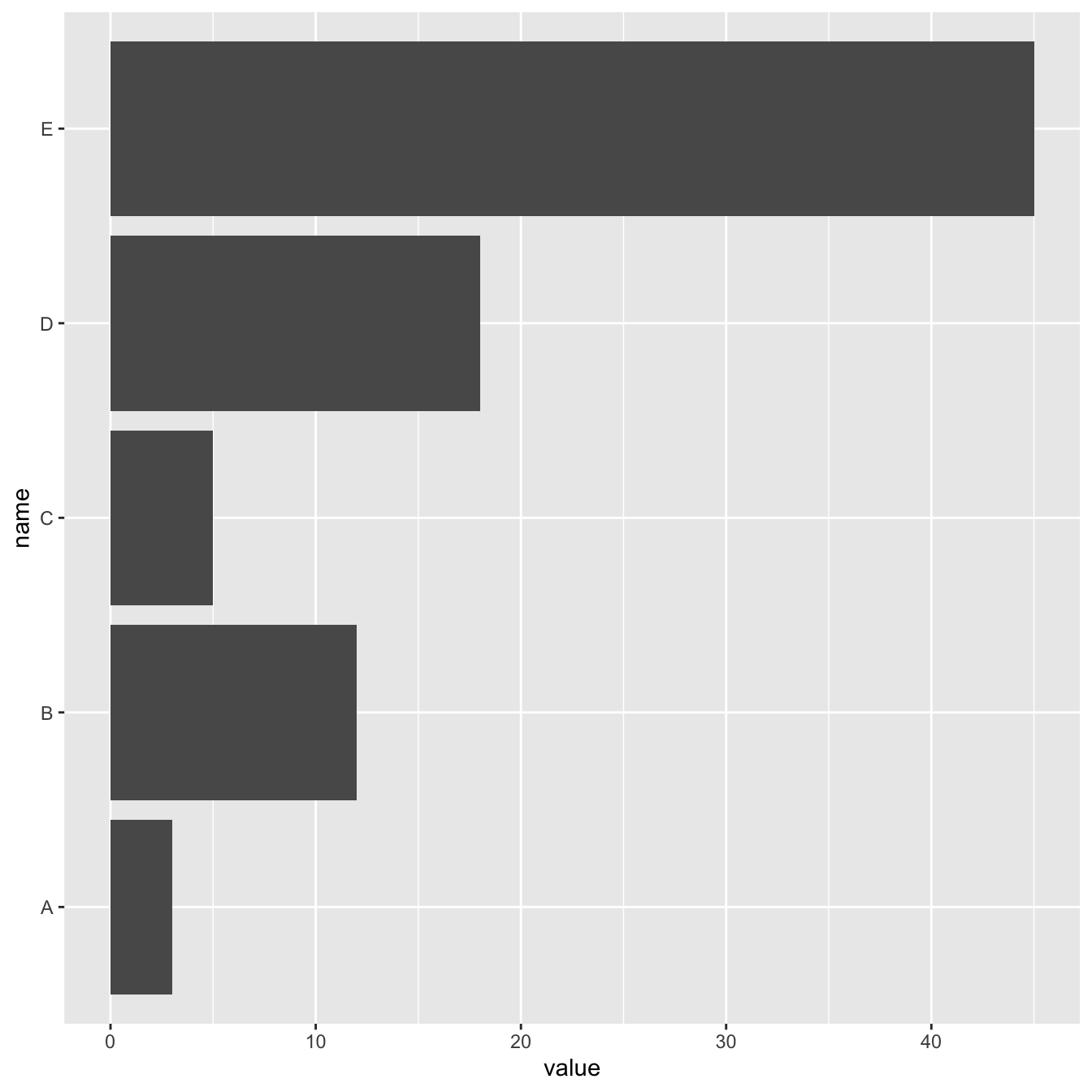``````# Load ggplot2
library(ggplot2)

# Create data
data <- data.frame(
name=c("A","B","C","D","E") ,
value=c(3,12,5,18,45)
)

# Barplot
ggplot(data, aes(x=name, y=value)) +
geom_bar(stat = "identity") +
coord_flip()``````

# Control bar width with `width`

The `width` argument of the `geom_bar()` function allows to control the bar width. It ranges between 0 and 1, 1 being full width.

See how this can be used to make bar charts with variable width.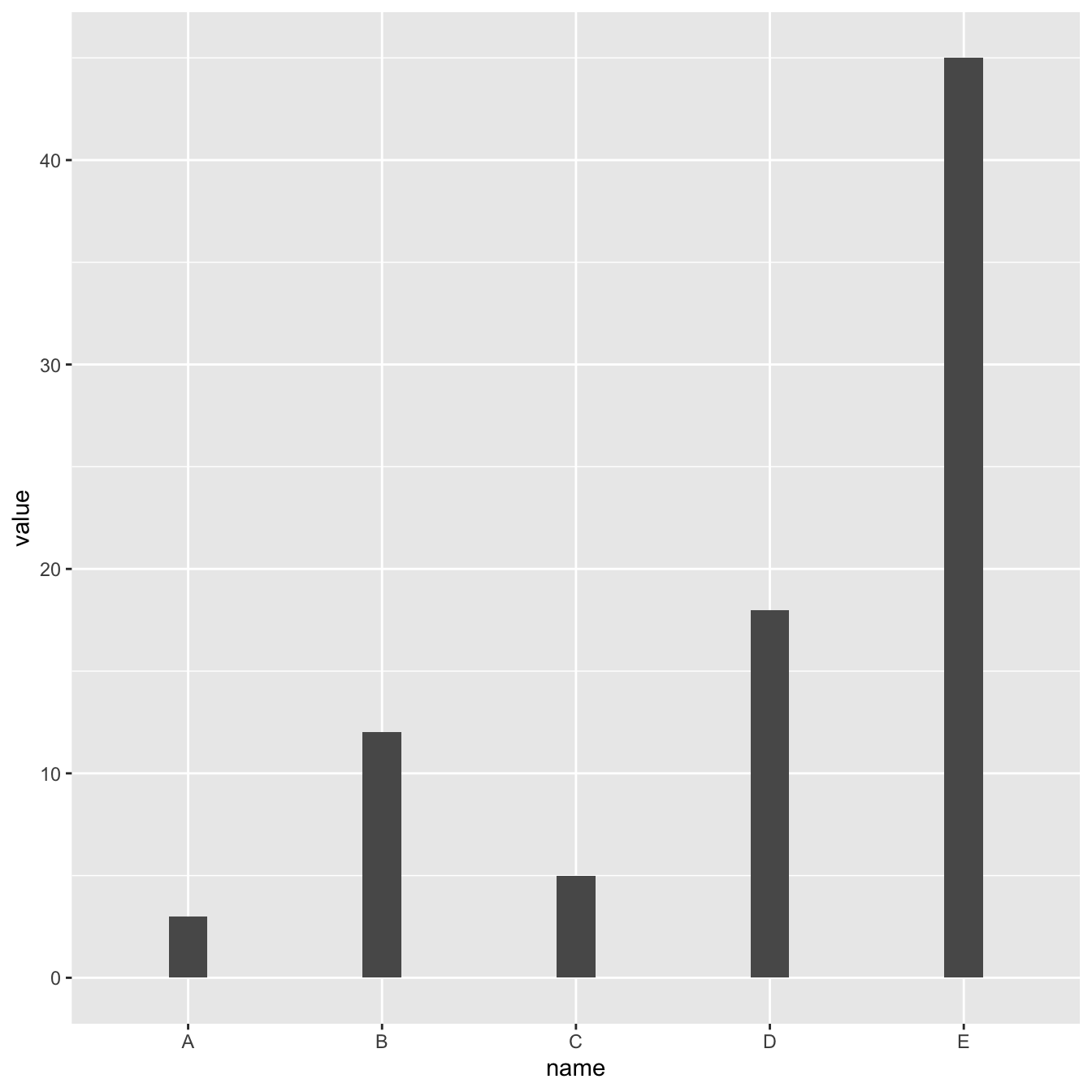``````# Load ggplot2
library(ggplot2)

# Create data
data <- data.frame(
name=c("A","B","C","D","E") ,
value=c(3,12,5,18,45)
)

# Barplot
ggplot(data, aes(x=name, y=value)) +
geom_bar(stat = "identity", width=0.2) ``````

# What’s next?

This post was an overview of ggplot2 barplots, showing the basic options of `geom_barplot()`. Visit the barplot section for more:

Related chart types

## Contact

This document is a work by Yan Holtz. Any feedback is highly encouraged. You can fill an issue on Github, drop me a message on Twitter, or send an email pasting yan.holtz.data with gmail.com.